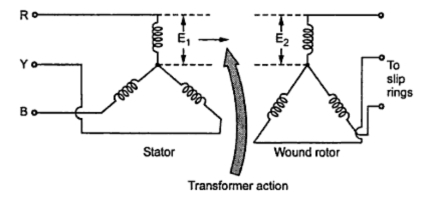### Induction Motor as a Transformer

We know that, transformer is a device in which two windings are magnetically coupled and when one winding is excited by a.c. supply of certain frequency, the e.m.f. gets induced in the second winding having same frequency as that of supply given to the first winding. The winding to which supply is given is called primary winding while winding in which e.m.f. gets induced is called secondary winding. The induction motor can be regarded as the transformer.
The difference is that the normal transformer is an alternating flux transformer while induction motor is rotating flux transformer. The normal transformer has no air gap as against this an induction motor has distinct air gap between its stator and rotor.
In an alternating flux transformer the frequency of induced e.m.f. and current in primary and secondary is always same. However in the induction motor frequency of e.m.f. and current on the stator side remains same but frequency of rotor e.m.f. and current depends on the slip and slip depends on load on the motor. So we have a variable frequency on the rotor side. But it is important to remember that at start when N = 0  the value of slip is unity (s = 1), then frequency of supply to the stator and of induced e.m.f. in the rotor is same. The effect of slip on the rotor parameters is already discussed in the previous section.
And last difference is that in case of the alternating flux transformer the entire energy present in the secondary circuit, is in the electrical form. As against this, in an induction motor part of its energy in the rotor circuit is in electrical form and the remaining part is converted into mechanical form.Fig. 1  Induction motor as a transformer

In general, an induction motor can be treated as a generalised transformer as shown in the Fig. 1. In this, the slip ring induction motor with star connected stator and rotor is shown.
So if            E1 = Stator e.m.f. per phase in volts.
E2 = Rotor induced e.m.f. per phase in volts at start when motor is at standstill.
Then according to general transformer there exists a fixed relation between E1 and E2 called transformer ratio.
...     At start when   N =0 , s =1
and we get,

Key Point : So if stator supply voltage is known and ratio of stator to rotor turns per phase is known then the rotor induced e.m.f. on standstill can be obtained.

1.2.